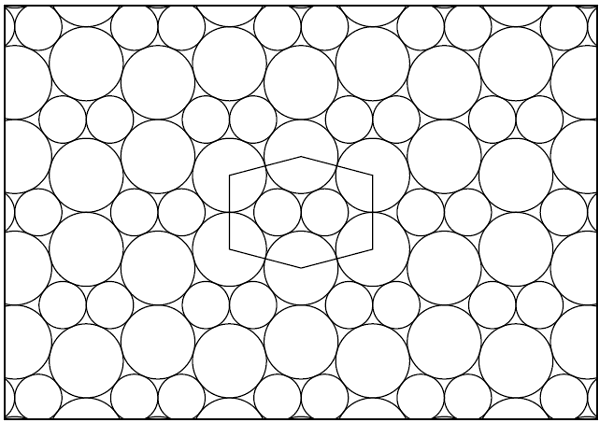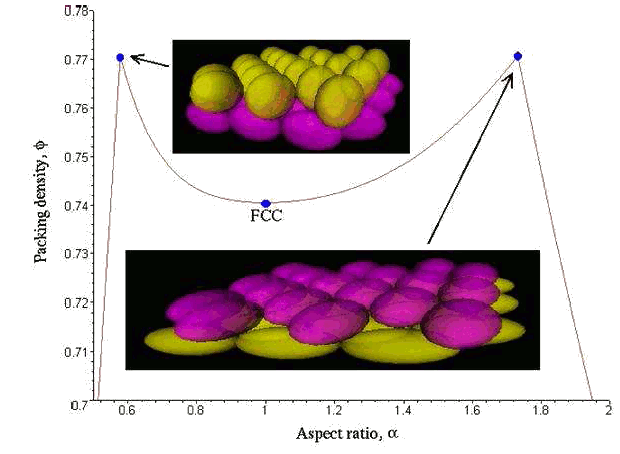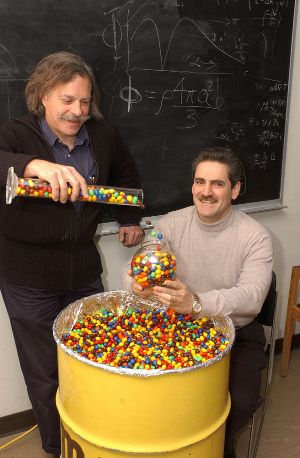### Packing

November 30, 2010

The arrangement of pennies on a plane that gives an optimal packing (most pennies per area) is so trivial that it's nearly instinctive. You arrange them such that their centers are on an hexagonal lattice. This optimal lattice packing of circles on a plane was proven mathematically by Carl Friedrich Gauss, and the resultant density is (π/2√3) = 0.9069. In 1940, László Fejes Tóth showed that this is the optimal packing, lattice or otherwise. We can still obtain compact packing when the coins are of different size, as shown in the figure. In this case, the coins have diameter 1 and the root of the equation, r4 - 10r2 - 8r + 9.Compact packing of two sizes of disks of radius 1 and 0.6375559772... Figure 1 of Ref. 2.

You wouldn't think that stepping up just one dimension from circles in a plane to spheres in a volume would cause much more of a problem. Greengrocers have been stacking fruit in nicely structured dense piles for centuries. However, proving that this arrangement, beloved by Nature and crystallographers as face-centered-cubic (FCC), is indeed optimal, or that there is a non-lattice packing that's more dense, is quite a problem. None other than Johannes Kepler first conjectured that this FCC lattice, also called cubic close-packing, was the optimal packing of spheres. Calculation shows that the packing is (π/√18) = 0.74048, which means that there's quite a lot of empty space between spheres, a fact that allows the creation of the interesting material, inverse opal. Thomas Hales produced a "proof" of this conjecture in 1998. His proof, which is much like the "proof" for the four color conjecture, used computer programs to examine every possible case of sphere packing. Checking such a proof is difficult, and even today mathematicians are not completely certain that the proof is correct. Even then, it would not be a proof in the usual sense of the word.

I've commented in several earlier articles that many scientific discoveries have been accidental. One packing discovery happened accidentally at Princeton University when a student was asked to experimentally measure random packing of a geometrical object. This object was M&M candy, which is an oblate spheroid. Surprisingly, a random assemblage of these was found to pack more densely than spheres, 68% as compared to 64% for random sphere packing.[4-6] The result was so surprising that Paul Chaikin, a professor of physics, repeated the experiment himself. He obtained the same result, and that led to a more detailed study. Other experiments showed that there was not much difference between spheres and M&Ms for lattice packing, only random packing. Computer simulations showed that a random stacking of ellipsoids, which are closer in shape to a sphere than the lenticular M&M, yields a greater density than a lattice stacking of spheres.Density of the laminate crystal packing of ellipsoids as a function of the aspect ratio, via arXiv, Ref. 6.

What might be happening in such random packing is the idea that the elongated particles are able to slide past each other to a better equilibrium position, while the spheres jam together. Of course, experiments are tedious, and computer simulations are easier. It was found that there are a greater number of contact points between neighboring particles for the higher packing densities. The number of contact points may depend on the number of directions a particle can move and pivot.

Paul Chaikin has moved to New York University, but Salvatore Torquato, a professor of chemistry and co-investigator on the original M&M project, is still at Princeton publishing numerous papers in this area.[7-12] He was an author of a Physical Review Letter on packing in 2000, so he's been active in this area for more than a decade. One interesting result is for the packing of the Platonic solids. A cube, of course, fills all space, but the densest packings of tetrahedra, icosahedra, dodecahedra, and octahedra were found to be 0.823, 0.836, 0.904, and 0.947. This is an interesting result, since the dodecahedra and icosahedra approximate a sphere to a high degree but pack much better.Paul Chaikin (left) and Salvatore Torquato (Princeton University)

During the original research project about a decade ago, Mars Inc., the M&M company, donated 125 pounds of almond M&M's; but men do not live by candy alone. Other funding came from the National Science Foundation, the NASA and the Petroleum Research Fund.### References:

Linked Keywords: penny; plane; synthetic a priori; hexagonal lattice; Carl Friedrich Gauss; László Fejes Tóth; root of a function; dimension; circle; sphere; volume; greengrocer; Nature; crystallography; crystallographers; face-centered-cubic; Johannes Kepler; Kepler conjecture; opal; Thomas Hales; four color conjecture; mathematician; serendipity; Princeton University; M&M candy; oblate spheroid; Paul Chaikin; physics; Computer simulation; New York University; Salvatore Torquato; Platonic solids; cube; tetrahedron; icosahedron; dodecahedron; octahedron; Mars Inc.; National Science Foundation; NASA; MathWorld; arXiv.